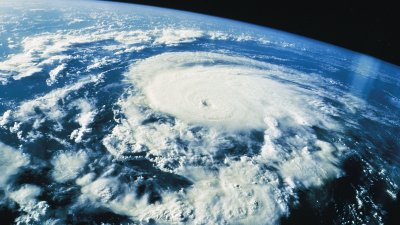# Fluid dynamics in mathematics

Fluid dynamics is the study of fluid motion (liquids, gases, and plasmas) and the forces exerted by the fluid on its surrounding. Check out our current research projects in fluid dynamics.

## Water waves (sloshing and wave energy harvesting)Shallow water sloshing and its implications for wave energy harvesting. Current work being led by Tom Bridges and Matthew Turner is focussing on the coupling between vessel motion and fluid motion in 3D. The vessel motion is modelled using the geometric mechanics framework for rigid body motion, and the fluid motion is modelled using a hierarchy of models from the shallow water equations, to irrotational 2D and 3D fluid motion, and up to the full inviscid Euler equations with exact representation of vorticity.

Also of interest is using ideas from complex analysis, in particular conformal mappings, to develop effecting numerical schemes to solve coupled sloshing problems. Utilizing the Schottky-Klein Prime function we can solve problems which involve multiply connected domains, such as vessels containing baffles.

## Vortex dynamicsUnderstanding the dynamics of vortices is important when considering things such as thermal and sedimentary transport in the oceans. Current research in this area led by Matthew Turner focuses on investigating how coherent vortices react to nonlinear perturbations, in particular looking at thresholds in parameter space for where these nonlinear perturbations persist for large times.

Also of interest currently is trying to predict when a pair of point vortices on the plane with some solid boundary propagate in a leap-frogging motion. Recent work has determined a numerical criteria, based upon vortex strengths and initial separation, for two vortices external to a circular cylinder. This work is now being extended to other doubly- and multiply connected domains.

## Hydrodynamic stabilityCurrent research in this area led Matthew Turner is looking at the absolute instability properties of spatially periodic flows, in particular a mixing layer with a spatially periodic bounding wall. The approach to determining the stability properties using Floquet theory in the spatial direction in order to form modal solutions and derive a dispersion relation for wave-like solutions. The global stability properties are then sought by looking for saddle points of the complex dispersion relation.

## Turbulent convection and mixing of fluidsMy means of techniques stemming from the theory of partial differential equations, harmonic analysis, and calculus of variation, led by Camilla Nobili, we aim at rigorously derive scaling laws for physically relevant quantities like heat transport, energy, and mixing degrees in turbulent flows.

Some key-topics are:

1. Bounds on Nusselt number in turbulent Rayleigh–Bénard convection and related systems
2. Role of boundary conditions and rotation in heat transport enhancement
3. Mixing estimates and convergence to the Batchelor scale
4. Anomalous dissipation.

## Geometric fluid mechanicsIan Roulstone and collaborators Tom Bridges (Surrey), V Rubtsov (Angers), Martin Wolf (Surrey) exploit ideas and results from contact and K\"ahler geometries in an attempt to understand the ubiquity and topology of vortex motion, and its role in many different fluid flows, from those in the atmosphere and ocean to high Reynolds number Navier-Stokes turbulence. This research agenda is a joint venture with the Fields, Strings, and Geometry (FSG) Group.

Current work is focused on the extraction of topological information from the Navier--Stokes equations, for incompressible flows in two and three spatial dimensions, by studying the geometry of so-called Lagrangian submanifolds. The curvature of these structures encapsulates the relationships between gradients of vorticity and strain. The connection with string theory arises because the Lagrangian submanifolds are embedded in four or six dimensional spaces, typically of K\"ahler or Calabi-Yau type.

This work forms the basis for two PhD projects, one in collaboration with the FSG group (Wolf) and another in collaboration with the School of Mathematics and Physics at Milano-Bicocca, under the auspices of an International Dual Doctorate (Bridges, Ortenzi).

• Email: